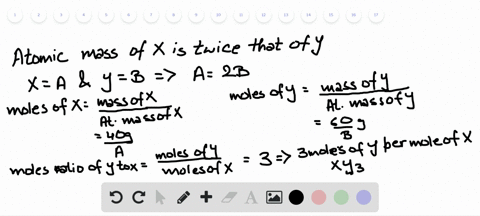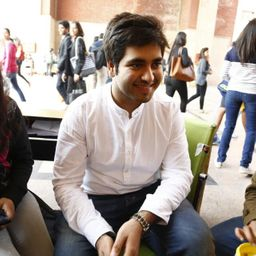### The elements X and Y form a compound that is 40% …

01:38University of New Mexico
Problem 147

A compound of molar mass 229 contains only carbon, hydrogen, iodine, and sulfur. Analysis shows that a sample of the compound contains 6 times as much carbon as hydrogen, by mass. Calculate the molecular formula of the compound.

the molecular formula of the compound is $\left[\mathrm{C}_{5} \mathrm{H}_{10} \mathrm{IS}\right]$

## Discussion

You must be signed in to discuss.
Miss B.
December 24, 2018
Problem55E -the substance KSCN is frequently used to test for iron in solution because of distinctive red forms when it is added. As a laboratory assistant, you need to prepare 1.00L Of 0.20M KSCN solution what mass in grams of KSCN is need?

## Video Transcript

from the question we came to know that the compound contains carbon, hydrogen, iodine and cell phone. The atomic mass for carbon is 12.1 hydrogen 1.8 Ionian 126.90 and cell phone. It is 32.7 We know the visual by masses off carbon to hydrogen is six Bhatia one. No, we need to calculate the number off. Hydrogen atom present for every carbon atom shown is the calculation over here on here. Hence, through this calculation, we are able to deter Mined that every carbon atom for every carbon atom, two hydrogen atoms are present. No, we need to calculate the mask off. One mole off ch two group. We know that the mass is mass for si Esto Mowlam Associates too is for the in 0.3 Grandpa mole, remember? Right. Remember the mullahs. Mass off compound is 229. Grandpa mole assumed that one ironing on one cell phone. Atom is present in one molecule off the compound. Now we need to calculate the mass off elements other than I Odin and sell for present inside the one mole of compound toe calculate. This shown is the calculation as it represented that this 70.3 gram per mole. Now we need to calculate the number off ch two group present inside the compound as according to the calculation, it comes out five. Therefore, the compound contains five cs two groups one idea an atom and once sulfur atom leading us to the molecular formula C five etched in I s.